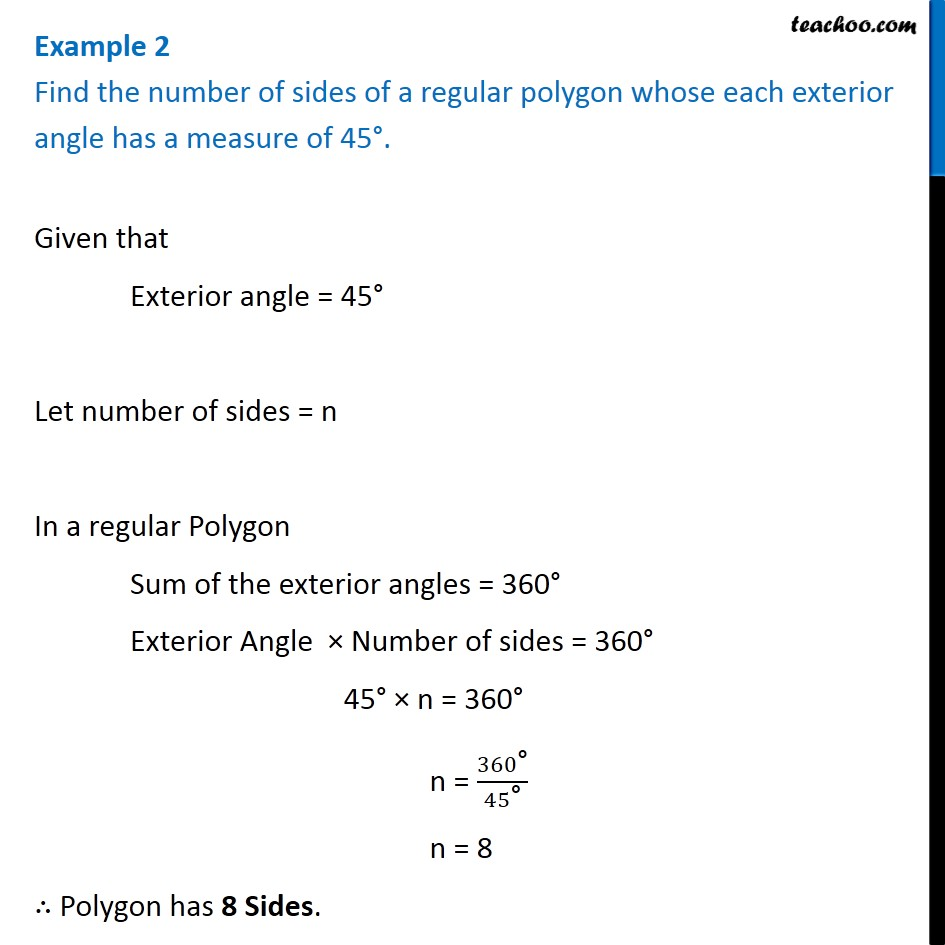Sum of Exterior Angles of PolygonsLearn in your speed, with individual attention - Teachoo Maths 1-on-1 Class

### Transcript

Example 2 Find the number of sides of a regular polygon whose each exterior angle has a measure of 45°. Given that Exterior angle = 45° Let number of sides = n In a regular Polygon Sum of the exterior angles = 360° Exterior Angle × Number of sides = 360° 45° × n = 360° n = 360"°" /45"°" n = 8 ∴ Polygon has 8 Sides.